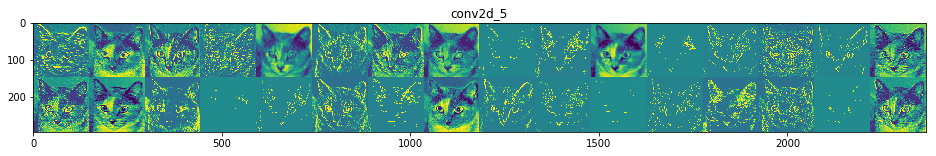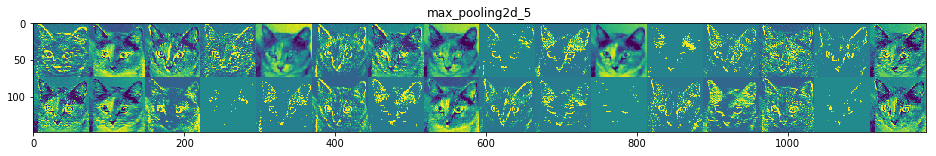In :
import keras
keras.__version__

Using TensorFlow backend.

Out:
'2.0.8'

# Visualizing what convnets learn¶

This notebook contains the code sample found in Chapter 5, Section 4 of Deep Learning with Python. Note that the original text features far more content, in particular further explanations and figures: in this notebook, you will only find source code and related comments.

It is often said that deep learning models are "black boxes", learning representations that are difficult to extract and present in a human-readable form. While this is partially true for certain types of deep learning models, it is definitely not true for convnets. The representations learned by convnets are highly amenable to visualization, in large part because they are representations of visual concepts. Since 2013, a wide array of techniques have been developed for visualizing and interpreting these representations. We won't survey all of them, but we will cover three of the most accessible and useful ones:

• Visualizing intermediate convnet outputs ("intermediate activations"). This is useful to understand how successive convnet layers transform their input, and to get a first idea of the meaning of individual convnet filters.
• Visualizing convnets filters. This is useful to understand precisely what visual pattern or concept each filter in a convnet is receptive to.
• Visualizing heatmaps of class activation in an image. This is useful to understand which part of an image where identified as belonging to a given class, and thus allows to localize objects in images.

For the first method -- activation visualization -- we will use the small convnet that we trained from scratch on the cat vs. dog classification problem two sections ago. For the next two methods, we will use the VGG16 model that we introduced in the previous section.

## Visualizing intermediate activations¶

Visualizing intermediate activations consists in displaying the feature maps that are output by various convolution and pooling layers in a network, given a certain input (the output of a layer is often called its "activation", the output of the activation function). This gives a view into how an input is decomposed unto the different filters learned by the network. These feature maps we want to visualize have 3 dimensions: width, height, and depth (channels). Each channel encodes relatively independent features, so the proper way to visualize these feature maps is by independently plotting the contents of every channel, as a 2D image. Let's start by loading the model that we saved in section 5.2:

In :
from keras.models import load_model

model.summary()  # As a reminder.

_________________________________________________________________
Layer (type)                 Output Shape              Param #
=================================================================
conv2d_5 (Conv2D)            (None, 148, 148, 32)      896
_________________________________________________________________
max_pooling2d_5 (MaxPooling2 (None, 74, 74, 32)        0
_________________________________________________________________
conv2d_6 (Conv2D)            (None, 72, 72, 64)        18496
_________________________________________________________________
max_pooling2d_6 (MaxPooling2 (None, 36, 36, 64)        0
_________________________________________________________________
conv2d_7 (Conv2D)            (None, 34, 34, 128)       73856
_________________________________________________________________
max_pooling2d_7 (MaxPooling2 (None, 17, 17, 128)       0
_________________________________________________________________
conv2d_8 (Conv2D)            (None, 15, 15, 128)       147584
_________________________________________________________________
max_pooling2d_8 (MaxPooling2 (None, 7, 7, 128)         0
_________________________________________________________________
flatten_2 (Flatten)          (None, 6272)              0
_________________________________________________________________
dropout_1 (Dropout)          (None, 6272)              0
_________________________________________________________________
dense_3 (Dense)              (None, 512)               3211776
_________________________________________________________________
dense_4 (Dense)              (None, 1)                 513
=================================================================
Total params: 3,453,121
Trainable params: 3,453,121
Non-trainable params: 0
_________________________________________________________________


This will be the input image we will use -- a picture of a cat, not part of images that the network was trained on:

In :
img_path = '/Users/fchollet/Downloads/cats_and_dogs_small/test/cats/cat.1700.jpg'

# We preprocess the image into a 4D tensor
from keras.preprocessing import image
import numpy as np

img_tensor = image.img_to_array(img)
img_tensor = np.expand_dims(img_tensor, axis=0)
# Remember that the model was trained on inputs
# that were preprocessed in the following way:
img_tensor /= 255.

# Its shape is (1, 150, 150, 3)
print(img_tensor.shape)

(1, 150, 150, 3)


Let's display our picture:

In :
import matplotlib.pyplot as plt

plt.imshow(img_tensor)
plt.show()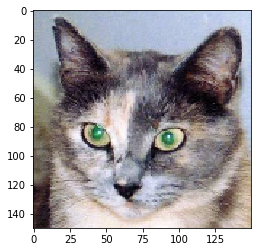In order to extract the feature maps we want to look at, we will create a Keras model that takes batches of images as input, and outputs the activations of all convolution and pooling layers. To do this, we will use the Keras class Model. A Model is instantiated using two arguments: an input tensor (or list of input tensors), and an output tensor (or list of output tensors). The resulting class is a Keras model, just like the Sequential models that you are familiar with, mapping the specified inputs to the specified outputs. What sets the Model class apart is that it allows for models with multiple outputs, unlike Sequential. For more information about the Model class, see Chapter 7, Section 1.

In :
from keras import models

# Extracts the outputs of the top 8 layers:
layer_outputs = [layer.output for layer in model.layers[:8]]
# Creates a model that will return these outputs, given the model input:
activation_model = models.Model(inputs=model.input, outputs=layer_outputs)


When fed an image input, this model returns the values of the layer activations in the original model. This is the first time you encounter a multi-output model in this book: until now the models you have seen only had exactly one input and one output. In the general case, a model could have any number of inputs and outputs. This one has one input and 8 outputs, one output per layer activation.

In :
# This will return a list of 5 Numpy arrays:
# one array per layer activation
activations = activation_model.predict(img_tensor)


For instance, this is the activation of the first convolution layer for our cat image input:

In :
first_layer_activation = activations
print(first_layer_activation.shape)

(1, 148, 148, 32)


It's a 148x148 feature map with 32 channels. Let's try visualizing the 3rd channel:

In :
import matplotlib.pyplot as plt

plt.matshow(first_layer_activation[0, :, :, 3], cmap='viridis')
plt.show()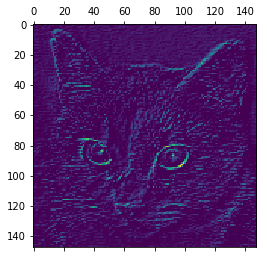This channel appears to encode a diagonal edge detector. Let's try the 30th channel -- but note that your own channels may vary, since the specific filters learned by convolution layers are not deterministic.

In :
plt.matshow(first_layer_activation[0, :, :, 30], cmap='viridis')
plt.show()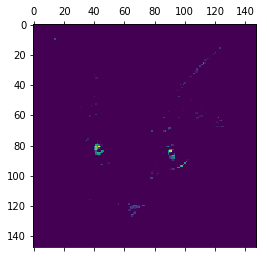This one looks like a "bright green dot" detector, useful to encode cat eyes. At this point, let's go and plot a complete visualization of all the activations in the network. We'll extract and plot every channel in each of our 8 activation maps, and we will stack the results in one big image tensor, with channels stacked side by side.

In :
import keras

# These are the names of the layers, so can have them as part of our plot
layer_names = []
for layer in model.layers[:8]:
layer_names.append(layer.name)

images_per_row = 16

# Now let's display our feature maps
for layer_name, layer_activation in zip(layer_names, activations):
# This is the number of features in the feature map
n_features = layer_activation.shape[-1]

# The feature map has shape (1, size, size, n_features)
size = layer_activation.shape

# We will tile the activation channels in this matrix
n_cols = n_features // images_per_row
display_grid = np.zeros((size * n_cols, images_per_row * size))

# We'll tile each filter into this big horizontal grid
for col in range(n_cols):
for row in range(images_per_row):
channel_image = layer_activation[0,
:, :,
col * images_per_row + row]
# Post-process the feature to make it visually palatable
channel_image -= channel_image.mean()
channel_image /= channel_image.std()
channel_image *= 64
channel_image += 128
channel_image = np.clip(channel_image, 0, 255).astype('uint8')
display_grid[col * size : (col + 1) * size,
row * size : (row + 1) * size] = channel_image

# Display the grid
scale = 1. / size
plt.figure(figsize=(scale * display_grid.shape,
scale * display_grid.shape))
plt.title(layer_name)
plt.grid(False)
plt.imshow(display_grid, aspect='auto', cmap='viridis')

plt.show()

/usr/local/lib/python3.5/dist-packages/ipykernel_launcher.py:30: RuntimeWarning: invalid value encountered in true_divide